# Simulation

Monday July 22, 2019

# Last week: Tidyverse II: tidyr

• tidyr is a package for manipulating the structure of data frames
• gather(): make wide data longer
• spread(): make long data wider
• unite() and separate(): combine or split columns
• dplyr has advanced functionality that mirrors SQL
• group_by(): create groups of rows according to a condition
• summarize(): apply computations across groups of rows
• *_join() where * = inner, left, right, or full: join two data frames together according to common values in certain columns, and * indicates how many rows to keep

# Part I

Simulation basics

# Why simulate?

R gives us unique access to great simulation tools (unique compared to other languages). Why simulate? Welcome to the 21st century! Two reasons:

• Often, simulations can be easier than hand calculations
• Often, simulations can be made more realistic than hand calculations

# Sampling from a given vector

To sample from a given vector, use sample()

sample(x = letters, size = 10) # Without replacement, the default
##   "w" "n" "j" "k" "l" "m" "x" "f" "u" "g"
sample(x = c(0,1), size = 10, replace = TRUE) # With replacement
##   1 0 1 0 0 0 1 1 1 0
sample(x = 10) # Arguments set as x=1:10, size=10, replace=FALSE
##    1  8  2  9 10  4  3  5  7  6

# Random number generation

To sample from a normal distribution, we have the utility functions:

• rnorm(): generate normal random variables
• pnorm(): normal distribution function, $$\Phi(x)=P(Z \leq x)$$
• dnorm(): normal density function, $$\phi(x)= \Phi'(x)$$
• qnorm(): normal quantile function, $$q(y)=\Phi^{-1}(y)$$, i.e., $$\Phi(q(y))=y$$

Replace “norm” with the name of another distribution, all the same functions apply. E.g., “t”, “exp”, “gamma”, “chisq”, “binom”, “pois”, etc.

# Random number examples

Standard normal random variables (mean 0 and variance 1)

n <- 200
z <- rnorm(n, mean = 0, sd = 1) # These are the defaults for mean, sd
mean(z)  # Check: sample mean is approximately 0
##  0.08054253
var(z)   # Check: sample variance is approximately 1
##  1.167726

# Estimated distribution function

To compute empirical cumulative distribution function (ECDF)—the standard estimator of the cumulative distribution function—use ecdf()

library(tidyverse)
ecdf_fun <- ecdf(z) # Create the ECDF
class(ecdf_fun) # It's a function!
##  "ecdf"     "stepfun"  "function"
ecdf_fun(0)
##  0.5
# We can plot it

gaussian_data <- data.frame(z = sort(z)) %>%
mutate(ecdf = ecdf_fun(z),
true = pnorm(z))

gaussian_data_lines <- gaussian_data %>%
gather("line", "value",
ecdf, true, -z) %>%
mutate(line = forcats::fct_recode(line,
Empirical = "ecdf",
Actual = "true"))

ggplot(gaussian_data_lines, aes(x = z, y = value, color = line)) +
geom_line() +
labs(y = "CDF",
title = "ECDF",
color = "")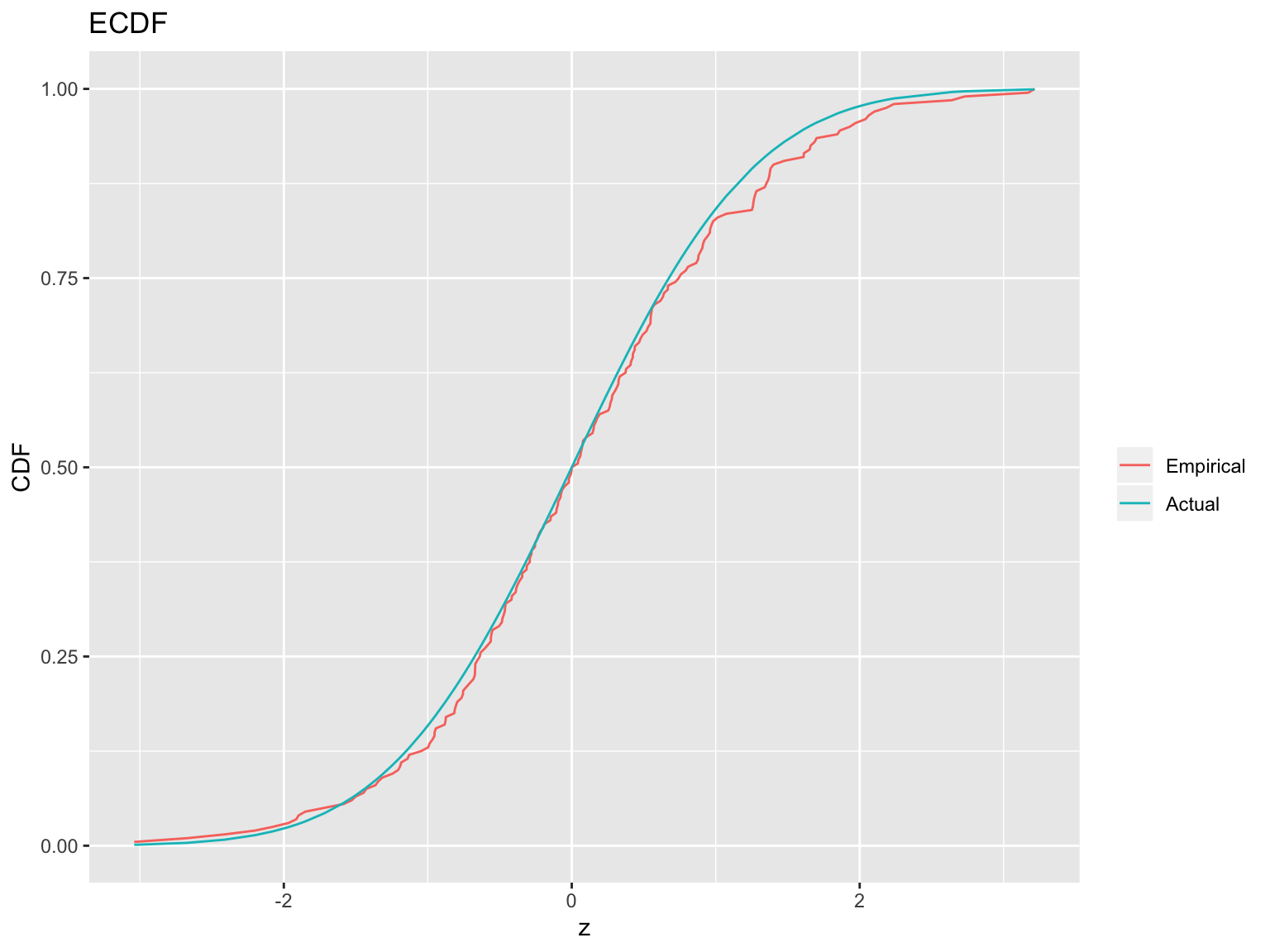# Estimated density function

To compute histogram —a basic estimator of the density based on binning—use hist()

hist_obj <- hist(z, breaks = 30, plot = FALSE) # base R function
class(hist_obj) # It's a list
##  "histogram"
hist_obj$breaks # These are the break points that were used ##  -3.2 -3.0 -2.8 -2.6 -2.4 -2.2 -2.0 -1.8 -1.6 -1.4 -1.2 -1.0 -0.8 -0.6 ##  -0.4 -0.2 0.0 0.2 0.4 0.6 0.8 1.0 1.2 1.4 1.6 1.8 2.0 2.2 ##  2.4 2.6 2.8 3.0 3.2 3.4 hist_obj$density # These are the estimated probabilities
##   0.025 0.000 0.025 0.025 0.000 0.050 0.100 0.025 0.125 0.125 0.125
##  0.300 0.375 0.350 0.450 0.400 0.350 0.300 0.425 0.225 0.325 0.050
##  0.300 0.050 0.150 0.100 0.100 0.025 0.000 0.050 0.000 0.025 0.025
# We can plot it
gaussian_data <- gaussian_data %>%
mutate(PDF = dnorm(z))

ggplot(gaussian_data, aes(x = z, y = ..density..)) +
geom_histogram(bins = 30,
fill = "lightblue",
col = "black") +
geom_line(aes(y = PDF)) +
labs(title = "True vs Empirical Distribution")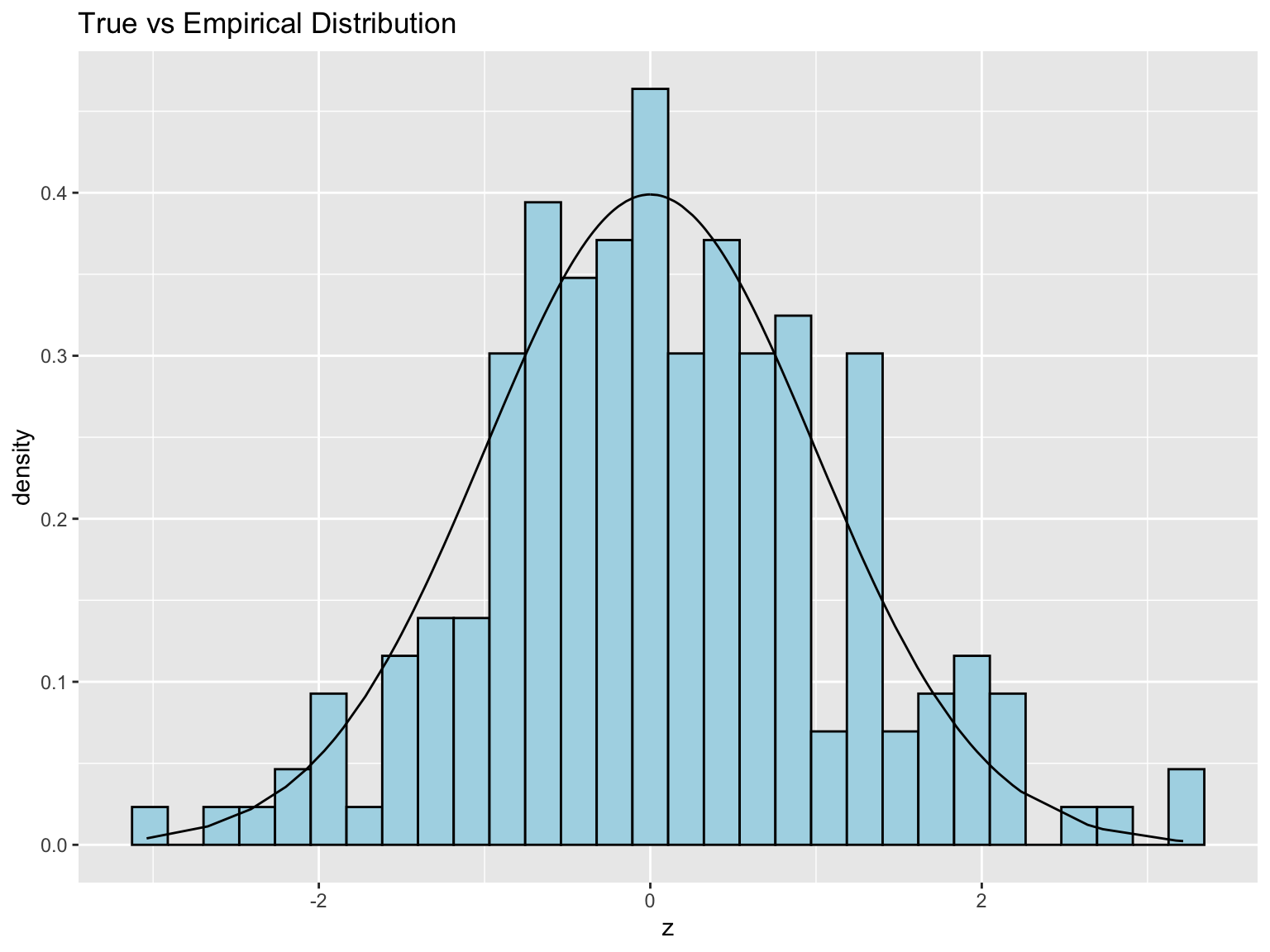hist_data <- data.frame(breaks = hist_obj$breaks[1:(length(hist_obj$breaks) - 1)] + .1,
density = hist_obj$density) ggplot(hist_data, aes(x = breaks, y = density)) + geom_histogram(stat = "identity") ## Warning: Ignoring unknown parameters: binwidth, bins, pad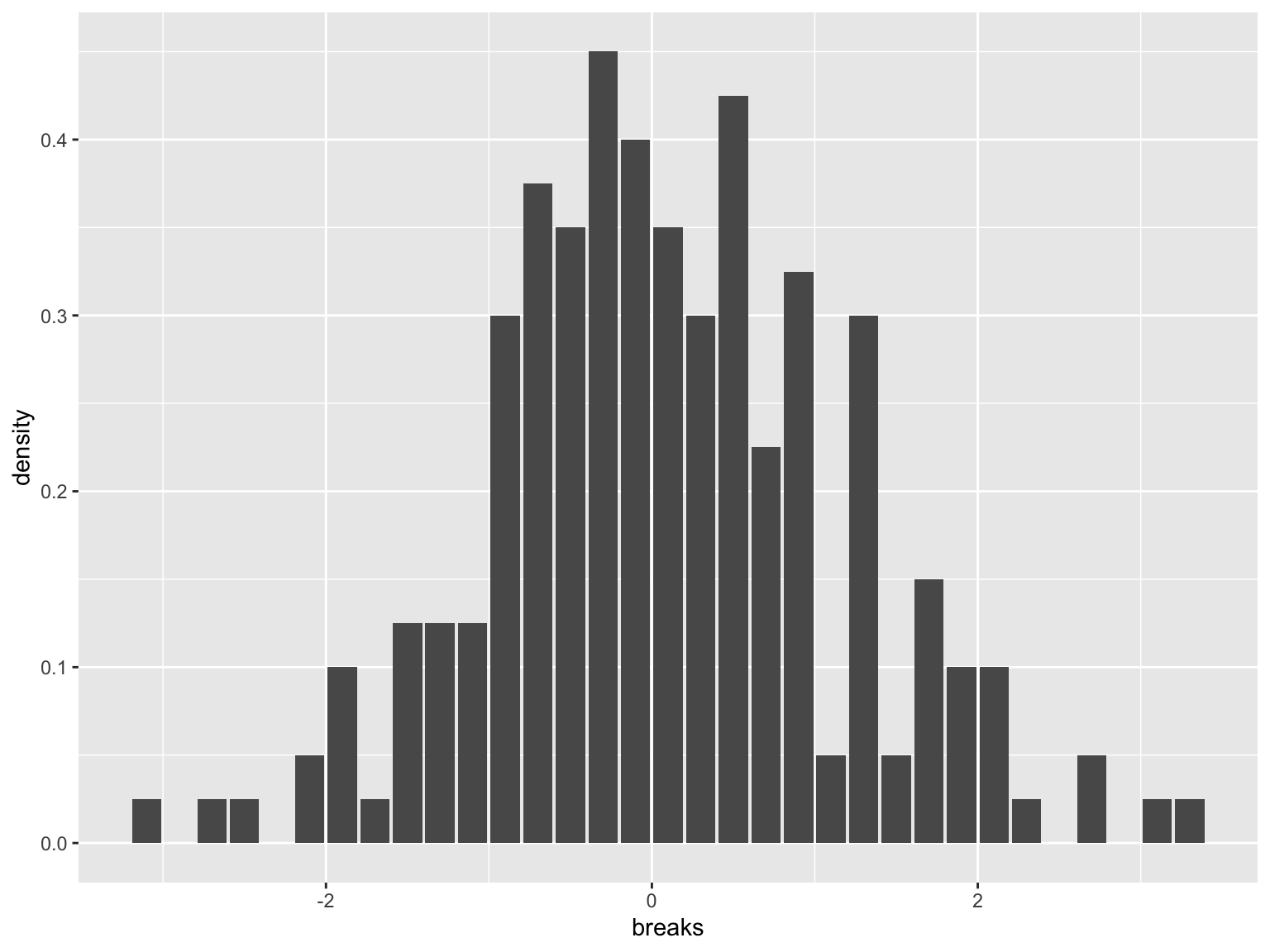# Tangent on estimation (did you know?) Interesting statistical facts: • When you have lots of data, the empirical distribution function will always be close to the actual distribution function (Glivenko-Cantelli, “Fundamental Theorem of Statistics”) • When you have lots of data, the histogram estimator is not always close to the density function, only when the underlying density is quite smooth • In general, density estimation is tricky, there are many estimators beyond histograms worth studying/knowing about; e.g., density() uses a kernel density estimator (gives a function) and you’ve already seen geom_density # Part II Pseudorandomness and seeds # Same function call, different results Not surprisingly, we get different draws each time we call rnorm() mean(rnorm(n)) ##  -0.06193826 mean(rnorm(n)) ##  -0.001027752 mean(rnorm(n)) ##  0.03538111 mean(rnorm(n)) ##  -0.1378349 # Is it really random? Random numbers generated in R (in any language) are not “truly” random; they are what we call pseudorandom • These are numbers generated by computer algorithms that very closely mimick “truly” random numbers • The study of such algorithms is an interesting research area in its own right! • The default algorithm in R (and in nearly all software languages) is called the “Mersenne Twister” • Type ?Random in your R console to read more about this (and to read how to change the algorithm used for pseudorandom number generation, which you should never really have to do, by the way) # Setting the random seed All pseudorandom number generators depend on what is called a seed value • This puts the random number generator in a well-defined “state”, so that the numbers it generates, from then on, will be reproducible • The seed is just an integer, and can be set with set.seed() • The reason we set it: so that when someone else runs our simulation code, they can see the same—albeit, still random—results that we do # Seed examples # Getting the same 5 random normals over and over set.seed(0); rnorm(5) ##  1.2629543 -0.3262334 1.3297993 1.2724293 0.4146414 set.seed(0); rnorm(5) ##  1.2629543 -0.3262334 1.3297993 1.2724293 0.4146414 set.seed(0); rnorm(5) ##  1.2629543 -0.3262334 1.3297993 1.2724293 0.4146414 # Different seeds, different numbers set.seed(1); rnorm(5) ##  -0.6264538 0.1836433 -0.8356286 1.5952808 0.3295078 set.seed(2); rnorm(5) ##  -0.89691455 0.18484918 1.58784533 -1.13037567 -0.08025176 set.seed(3); rnorm(5) ##  -0.9619334 -0.2925257 0.2587882 -1.1521319 0.1957828 # Each time the seed is set, the same sequence follows (indefinitely) set.seed(0); rnorm(3); rnorm(2); rnorm(1) ##  1.2629543 -0.3262334 1.3297993 ##  1.2724293 0.4146414 ##  -1.53995 set.seed(0); rnorm(3); rnorm(2); rnorm(1) ##  1.2629543 -0.3262334 1.3297993 ##  1.2724293 0.4146414 ##  -1.53995 set.seed(0); rnorm(3); rnorm(2); rnorm(1) ##  1.2629543 -0.3262334 1.3297993 ##  1.2724293 0.4146414 ##  -1.53995 # Part III Iteration and simulation # Drug effect model • Let’s start with a motivating example: suppose we had a model for the way a drug affected certain patients • All patients will undergo chemotherapy. We believe those who aren’t given the drug experience a reduction in tumor size of percentage $X_{\mathrm{no\,drug}} \sim 100 \cdot \mathrm{Exp}(\mathrm{mean}=R), \;\;\; R \sim \mathrm{Unif}(0,1)$ • And those who were given the drug experience a reduction in tumor size of percentage $X_{\mathrm{drug}} \sim 100 \cdot \mathrm{Exp}(\mathrm{mean}=2)$ (Here $$\mathrm{Exp}$$ denotes the exponential distribution, and $$\mathrm{Unif}$$ the uniform distribution) # What would you do? What would you do if you had such a model, and your scientist collaborators asked you: how many patients would we need to have in each group (drug, no drug), in order to reliably see that the average reduction in tumor size is large? • Answer used to be: get out your pen and paper, make some approximations • Answer is now: simulate from the model, no approximations required! # So, let’s simulate! # Simulate, supposing 60 subjects in each group set.seed(0) n <- 60 mu_drug <- 2 mu_nodrug <- runif(n, min = 0, max = 1) x_drug <- 100 * rexp(n, rate = 1/mu_drug) x_nodrug <- 100 * rexp(n, rate = 1/mu_nodrug) sim_data <- data.frame(x = c(x_drug, x_nodrug), class = factor(rep(c("Drug","No Drug"), each = 60))) sim_data %>% ggplot(aes(x = x, y = ..density.., color = class)) + geom_histogram(aes(fill = class), alpha = .5, color = "black", position = "identity") + geom_density(aes(color = class)) ## stat_bin() using bins = 30. Pick better value with binwidth.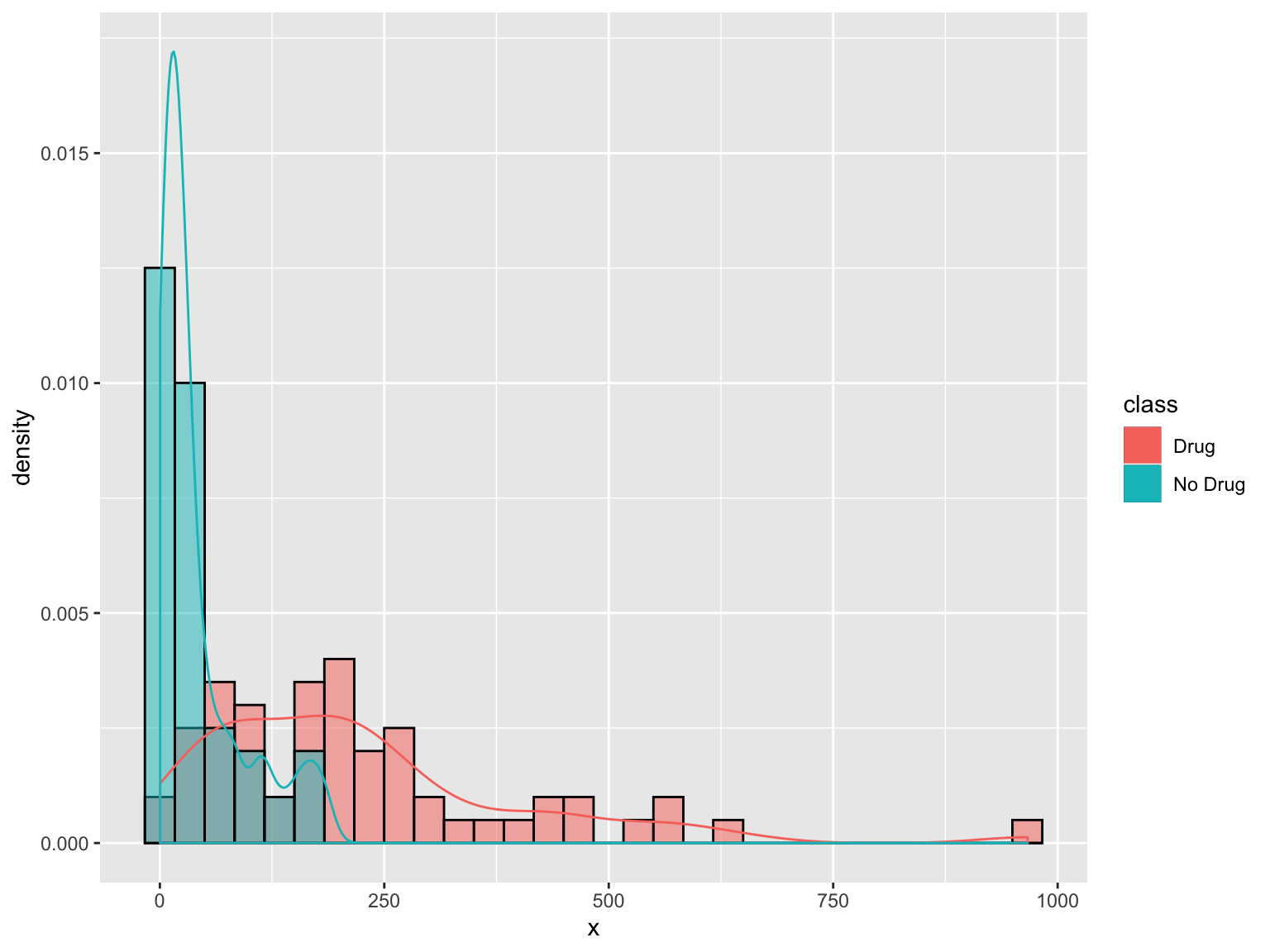# Kernel Density Estimates • When we use geom_density we are really getting a kernel density estimate - which can be thought of as adding little globs of honey centered at each point. • we can change the “spread” of each individual glob of honey, etc If we just want to calculate the a density function we can change the “spread” by changing the bandwidth (bw). For ggplot we can access this by using the stat_density instead of geom_density: library(gridExtra) gg_vis <- list() for (bw in c(5,10,30)) { gg_vis[[as.character(bw)]] <- sim_data %>% ggplot(aes(x = x, color = class)) + geom_density(aes(color = class), bw = bw) + geom_rug() + labs(title = bw) } grid.arrange(grobs = gg_vis, ncol = 1)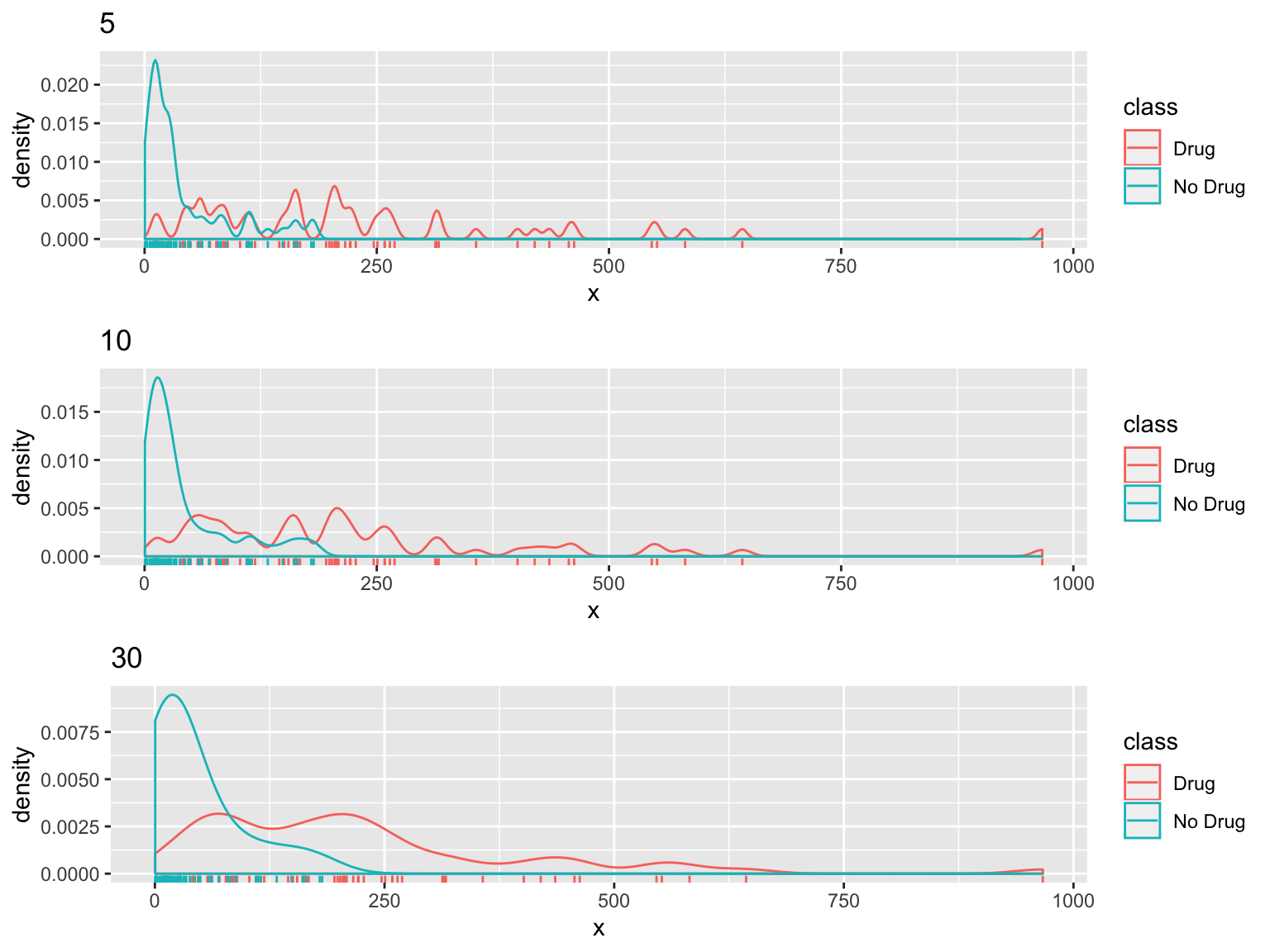# Repetition and reproducibility • One single simulation is not always trustworthy (depends on the situation, of course) • In general, simulations should be repeated and aggregate results reported—requires iteration! • To make random number draws reproducible, we must set the seed with set.seed() • More than this, to make simulation results reproducible, we need to follow good programming practices • Gold standard: any time you show a simulation result (a figure, a table, etc.), you have code that can be run (by anyone) to produce exactly the same result # Iteration and simulation (and functions): good friends • Writing a function to complete a single run of your simulation is often very helpful • This allows the simulation itself to be intricate (e.g., intricate steps, several simulation parameters), but makes running the simulation simple • Then you can use iteration to run your simulation over and over again • Good design practice: write another function for this last part (running your simulation many times) # Code sketch Consider the code below for a generic simulation. Think about how you would frame this for the drug effect example, which you’ll revisit in lab # Function to do one simulation run one_sim <- function(param1, param2 = value2, param3 = value3) { # Possibly intricate simulation code goes here } # Function to do repeated simulation runs rep_sim <- function(nreps, param1, param2 = value2, param3 = value3, seed = NULL) { # Set the seed, if we need to if(!is.null(seed)) set.seed(seed) # Run the simulation over and over sim_objs <- vector(length = nreps, mode = "list") for (r in 1:nreps) { sim_objs[r] <- one_sim(param1, param2, param3) } # Aggregate the results somehow, and then return something } # Part IV Reading in and writing out data # Saving results Sometimes simulations take a long time to run, and we want to save intermediate or final output, for quick reference later There two different ways of saving things from R (there are more than two, but here are two useful ones): • saveRDS(): allows us to save single R objects (like a vector, matrix, list, etc.), in (say) .rds format. E.g., saveRDS(my_mat, file = "my_matrix.rds") • save(): allows us to save any number of R objects in (say) .rdata format. E.g., save(mat_x, mat_y, list_z, file="my_objects.rdata") Note: there is a big difference between how these two treat variable names # Loading results Corresponding to the two different ways of saving, we have two ways of loading things into R: • readRDS(): allows us to load an object that has been saved by savedRDS(), and assign a new variable name. E.g., my_new_mat <- readRDS("my_matrix.rds") • load(): allows us to load all objects that have been saved through save(), according to their original variables names. E.g., load("my_objects.rdata") # Commentary on R based saving and loading • readRDS(), saveRDS(): functions for reading/writing single R objects from/to a file • load(), save(): functions for reading/writing any number of R objects from/to a file Advantage: these can be a lot more memory efficient than other tools Disadvantage: they’re limited in scope in the sense that they can only communicate with R # Reading in data from the outside All along, we’ve already been reading in data from the outside, using: • readLines(): reading in lines of text from a file or webpage; returns vector of strings • read.table(): read in a data table from a file or webpage; returns data frame • read.csv(): like the above, but assumes comma separated data; returns data frame This week we’ll focus on read.table(), read.csv() and their counterparts write.table(), write.csv(), respectively # read.table(), read.csv() Have a table full of data, just not in the R file format? Then read.table() is the function for you. It works as in: • read.table(file = file.name, sep = " "), to read data from a local file on your computer called file.name, assuming (say) space separated data • read.table(file = webpage_link, sep = "\t"), to read data from a webpage up at webpage_link, assuming (say) tab separated data The function read.csv() is just a shortcut for using read.table() with sep=",". (But note: these two actually differ on some of the default inputs!) # Helpful input arguments The following inputs apply to either read.table() or read.csv() (though these two functions actually have different default inputs in general—e.g., header defaults to TRUE in read.table() but FALSE in read.csv()) • header: boolean, TRUE is the first line should be interpreted as column names • sep: string, specifies what separates the entries; empty string “” is interpreted to mean any white space • check.names: boolean, if FALSE, don’t update names of columns to be more $ friendly
• stringsAsFactors: boolean, if FALSE - leave strings a strings (if TRUE converts them to factors)

Other helpful inputs: skip, row.names, col.names. You can read about them in the help file for read.table()

# Example of reading in data

shark_attacks <- read.table(
file="https://raw.githubusercontent.com/benjaminleroy/36-350-summer-data/master/Week2/shark-attacks-clean.csv",
sep = ",", header = TRUE)
head(shark_attacks)
##   X  Case.Number       Date       Type   Country              Area
## 1 1 2016.09.18.c 2016-09-18 Unprovoked       USA           Florida
## 2 2 2016.09.18.b 2016-09-18 Unprovoked       USA           Florida
## 3 3 2016.09.18.a 2016-09-18 Unprovoked       USA           Florida
## 4 4   2016.09.17 2016-09-17 Unprovoked AUSTRALIA          Victoria
## 5 5   2016.09.15 2016-09-16 Unprovoked AUSTRALIA          Victoria
## 6 6 2016.09.15.R 2016-09-15       Boat AUSTRALIA Western Australia
##                           Location Activity                   Name Sex Age
## 1 New Smyrna Beach, Volusia County  Surfing                   male   M  24
## 2 New Smyrna Beach, Volusia County  Surfing         Chucky Luciano   M  74
## 3 New Smyrna Beach, Volusia County  Surfing                   male   M  85
## 4                 Thirteenth Beach  Surfing        Rory Angiolella   M  -5
## 5                      Bells Beach  Surfing                   male   M  -5
## 6                          Bunbury  Fishing Occupant: Ben Stratton   U  -5
##                                     Injury  Time   Species
## 1                    Minor injury to thigh 13h00      <NA>
## 2                     Lacerations to hands 11h00      <NA>
## 3                 Lacerations to lower leg 10h43      <NA>
## 4             Struck by fin on chest & leg  <NA>      <NA>
## 5    No injury: Knocked off board by shark  <NA> 2 m shark
## 6 Shark rammed boat. No injury to occupant  <NA>      <NA>
##        Investigator.or.Source Fatal SharkLength
## 1 Orlando Sentinel, 9/19/2016     N          10
## 2 Orlando Sentinel, 9/19/2016     N           9
## 3 Orlando Sentinel, 9/19/2016     N           5
## 4          The Age, 9/18/2016     N           8
## 5          The Age, 9/16/2016     N           9
## 6  West Australian, 9/15/2016     N          10
sapply(shark_attacks, class)
##                      X            Case.Number                   Date
##              "integer"               "factor"               "factor"
##                   Type                Country                   Area
##               "factor"               "factor"               "factor"
##               Location               Activity                   Name
##               "factor"               "factor"               "factor"
##                    Sex                    Age                 Injury
##               "factor"              "integer"               "factor"
##                   Time                Species Investigator.or.Source
##               "factor"               "factor"               "factor"
##                  Fatal            SharkLength
##               "factor"              "integer"

# write.table(), write.csv()

To write a data frame (or matrix) to a text file, use write.table() or write.csv(). These are the counterparts to read.table() and read.csv(), and they work as in:

• write.table(my_df, file = "my_df.txt", sep = " ", quote = FALSE), to write my_df to the text file “my_df.txt” (to be created in your working directory), with (say) space separation, and no quotes around the entries
• write.csv(my_df, file="my_df.csv", quote = FALSE), to write my_dat to the text file “my_df.csv” (to be created in your working directory), with comma separation, and no quotes

Note that quote = FALSE, signifying that no quotes should be put around the printed data entries, seems always preferable (default is quote = TRUE). Also, setting row.names = FALSE and col.names = FALSE will disable the printing of row and column names (defaults are row.names = TRUE and col.names = TRUE)

# Summary

• Running simulations is an integral part of being a statistician in the 21st century
• R provides us with a utility functions for simulations from a wide variety of distributions
• To make your simulation results reproducible, you must set the seed, using set.seed()
• There is a natural connection between iteration, functions, and simulations
• Saving and loading results can be done in two formats: rds and rdata formats
• Read in data from a previous R session with readRDS(), load()
• Read in data from the outside with read.table(), read.csv()
• Can sometimes be tricky to get arguments right in read.table(), read.csv(); helps sometimes take a look at the original data files to see their structure